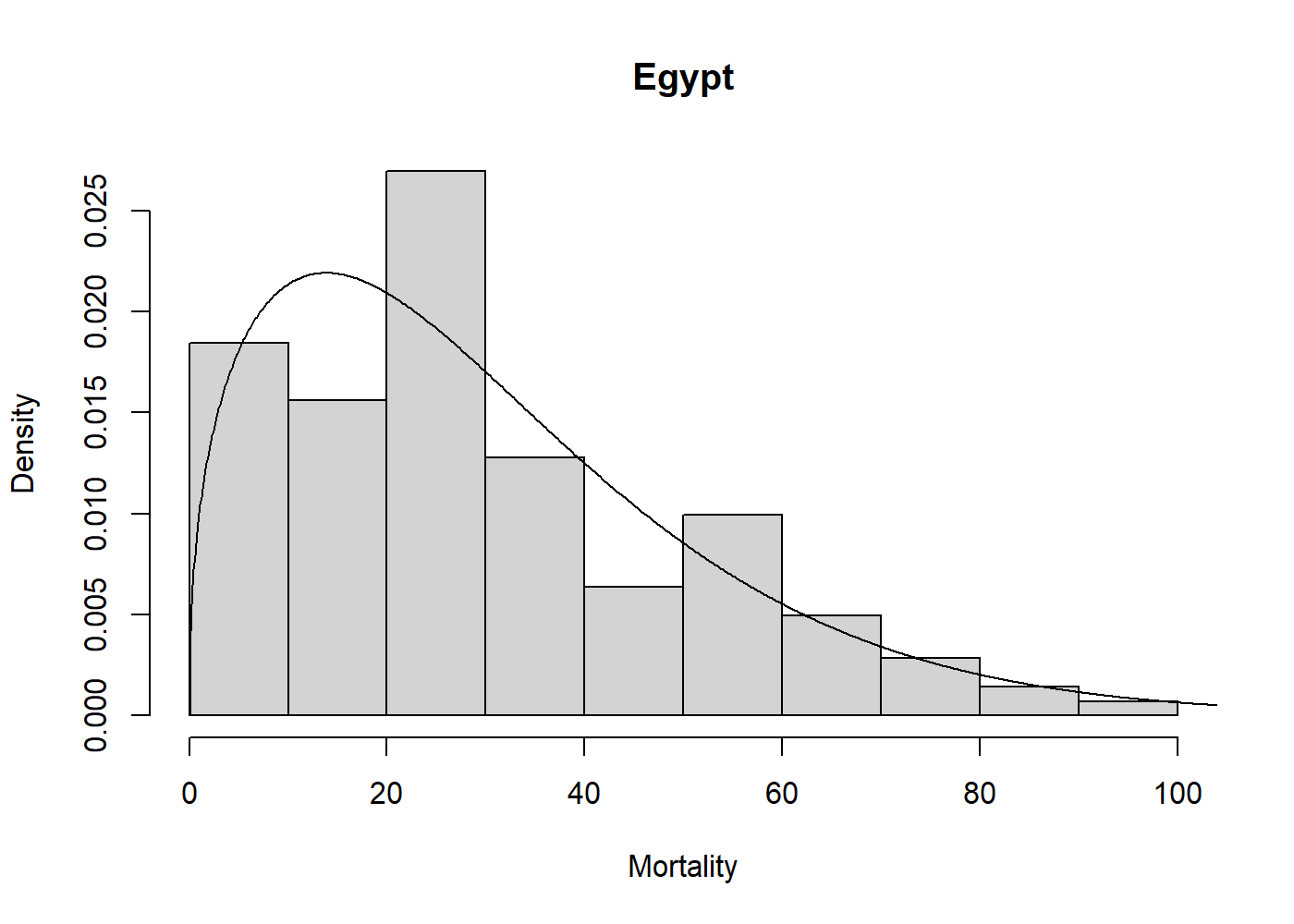# univariateML## Overview

`univariateML` is an `R`-package for user-friendly maximum likelihood estimation of a selection of parametric univariate densities. In addition to basic estimation capabilities, this package support visualization through `plot` and `qqmlplot`, model selection by `AIC` and `BIC`, confidence sets through the parametric bootstrap with `bootstrapml`, and convenience functions such as the density, distribution function, quantile function, and random sampling at the estimated distribution parameters.

## Installation

Use the following command from inside `R` to install from CRAN.

``install.packages("univariateML")``

Or install the development version from Github.

``````# install.packages("devtools")
devtools::install_github("JonasMoss/univariateML")``````

## Usage

The core of `univariateML` are the `ml***` functions, where `***` is a distribution suffix such as `norm`, `gamma`, or `weibull`.

``````library("univariateML")
mlweibull(egypt\$age)
#> Maximum likelihood estimates for the Weibull model
#>  shape   scale
#>  1.404  33.564``````

Now we can visually assess the fit of the Weibull model to the `egypt` data with a plot.

``````hist(egypt\$age, freq = FALSE, xlab = "Mortality", main = "Egypt")
lines(mlweibull(egypt\$age))``````## Supported densities

Name univariateML function Package
Cauchy distribution `mlcauchy` stats
Gumbel distribution `mlgumbel` extraDistr
Laplace distribution `mllaplace` extraDistr
Logistic distribution `mllogis` stats
Normal distribution `mlnorm` stats
Student t distribution `mlstd` fGarch
Generalized Error distribution `mlged` fGarch
Skew Normal distribution `mlsnorm` fGarch
Skew Student t distribution `mlsstd` fGarch
Skew Generalized Error distribution `mlsged` fGarch
Beta prime distribution `mlbetapr` extraDistr
Exponential distribution `mlexp` stats
Gamma distribution `mlgamma` stats
Inverse gamma distribution `mlinvgamma` extraDistr
Inverse Gaussian distribution `mlinvgauss` actuar
Inverse Weibull distribution `mlinvweibull` actuar
Log-logistic distribution `mlllogis` actuar
Log-normal distribution `mllnorm` stats
Lomax distribution `mllomax` extraDistr
Rayleigh distribution `mlrayleigh` extraDistr
Weibull distribution `mlweibull` stats
Log-gamma distribution `mllgamma` actuar
Pareto distribution `mlpareto` extraDistr
Beta distribution `mlbeta` stats
Kumaraswamy distribution `mlkumar` extraDistr
Logit-normal `mllogitnorm` logitnorm
Uniform distribution `mlunif` stats
Power distribution `mlpower` extraDistr

## Implementations

Analytic formulae for the maximum likelihood estimates are used whenever they exist. Most `ml***` functions without analytic solutions have a custom made Newton-Raphson solver. These can be much faster than a naïve solution using `nlm` or `optim`. For example, `mlbeta` has a large speedup over the naïve solution using `nlm`.

``````# install.packages("microbenchmark")
set.seed(313)
x <- rbeta(500, 2, 7)

microbenchmark::microbenchmark(
univariateML = univariateML::mlbeta(x),
naive = nlm(function(p) -sum(dbeta(x, p, p, log = TRUE)), p = c(1, 1)))
#> Unit: microseconds
#>          expr     min       lq      mean   median       uq     max neval
#>  univariateML   259.2   348.75   557.959   447.05   536.40  5103.5   100
#>         naive 15349.1 15978.35 16955.165 16365.45 17082.25 48941.4   100``````

The maximum likelihood estimators in this package have all been subject to testing, see the `tests` folder for details.

## Documentation

For an overview of the package and its features see the overview vignette. For an illustration of how this package can make an otherwise long and laborious process much simpler, see the copula vignette.

## How to Contribute or Get Help

Please read `CONTRIBUTING.md` for details about how to contribute or get help.